# Multiplying: 3 digits times 1 digit | Multiplication and division | Arithmetic | Khan Academy

##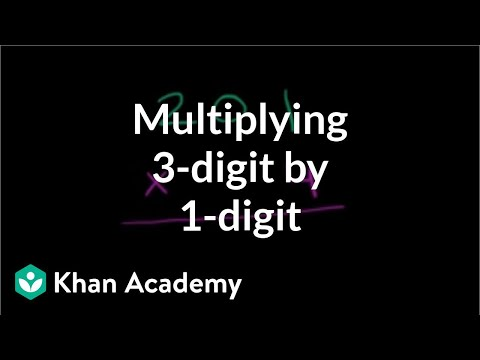By Khan Academy

How about this example? We're going to multiply a 3 digit number by a 1 digit number. Again, no carrying involved.# Example 3: Multiplying a monomial by a polynomial | Algebra I | Khan Academy

##By Khan Academy

Example 3: Multiplying a monomial by a polynomial | Algebra I | Khan Academy# Multiplying decimals 3

##By Khan Academy

Sometimes multiplying really small decimals (with all those zeros!) can be a little intimidating. Watch as we show you a handy trick to simplify these problems and solve them.# Multiplying decimals 3

##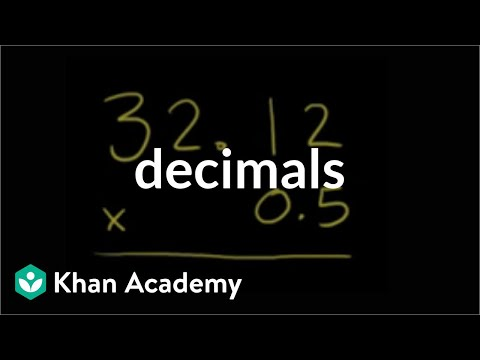By Khan Academy

Multiplying decimals? Try multiplying without the decimals first, them add them back in. We'll show you.# [3.MD.7b-1.0] Multiply to find Area - Common Core Standard

##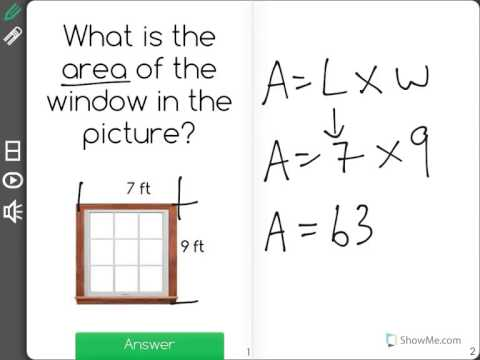By Freckle education

Multiply side lengths to find areas of rectangles with whole-number side lengths in the context of solving real world and mathematical problems# Multiplying Single Digit Numbers - Arrays & the Commutative Property (3.OA.1, 3.OA.3)

##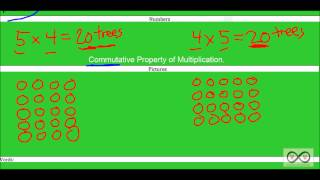By Worksheets and Walkthroughs

This video walkthrough guides the students through two single digit multiplication word problems. Solutions are represented as arrays while proving the commutative property.# Multiplying: 3 digits times 1 digit (with carrying) | Arithmetic | Khan Academy

##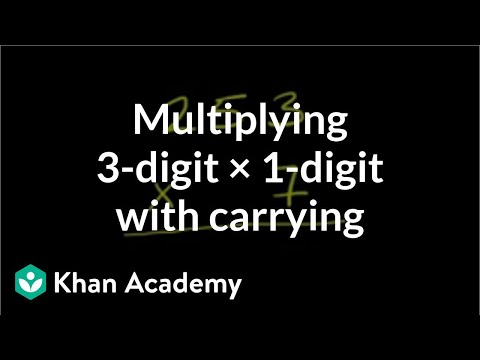By Khan Academy

We're carrying numbers in this example. Everyone needs a hand now and then, right?# Multiplication and Division Fact Families

##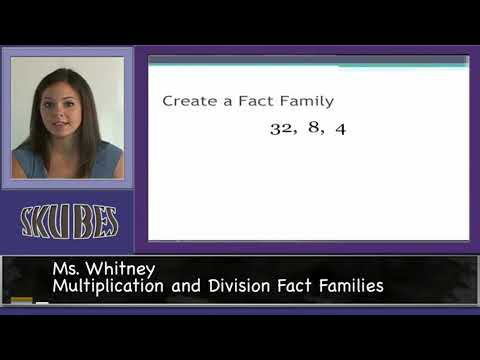By Skubes ed

3.OA.7 Fluently multiply and divide within 100, using strategies such as the relationship between multiplication and division (e.g., knowing that 8 × 5 = 40, one knows 40 ÷ 5 = 8) or properties# Multiplying mixed numbers

##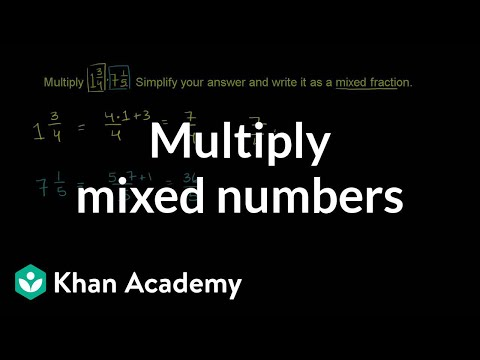By Khan Academy

Learn how to multiply two mixed numbers. The example used in this video is 1 and 3/4 multiplied by 7 and 1/5.# Multiplying mixed numbers

##By Khan Academy

Learn how to multiply two mixed numbers. The example used in this video is 1 and 3/4 multiplied by 7 and 1/5.# Multiplying mixed numbers

##By Khan Academy

Learn how to multiply two mixed numbers. The example used in this video is 1 and 3/4 multiplied by 7 and 1/5.# Multiplication 7: Old video giving more examples

##By Khan Academy

This video shows examples of multiplying a 3 digit number times a 2 digit number and an example of multiplying a 3 digit number times a 3 digit number. The instructor walks you through the process step by step on a chalkboard.# Multiples of Numbers - MathHelp.com - Math Help

##By yourteachermathhelp

In this lesson students learn that multiples of a number come from multiplying the given number by whole numbers. For example 3 x 0 = 0 3# [3.MD.7B] Multiply to find Area

##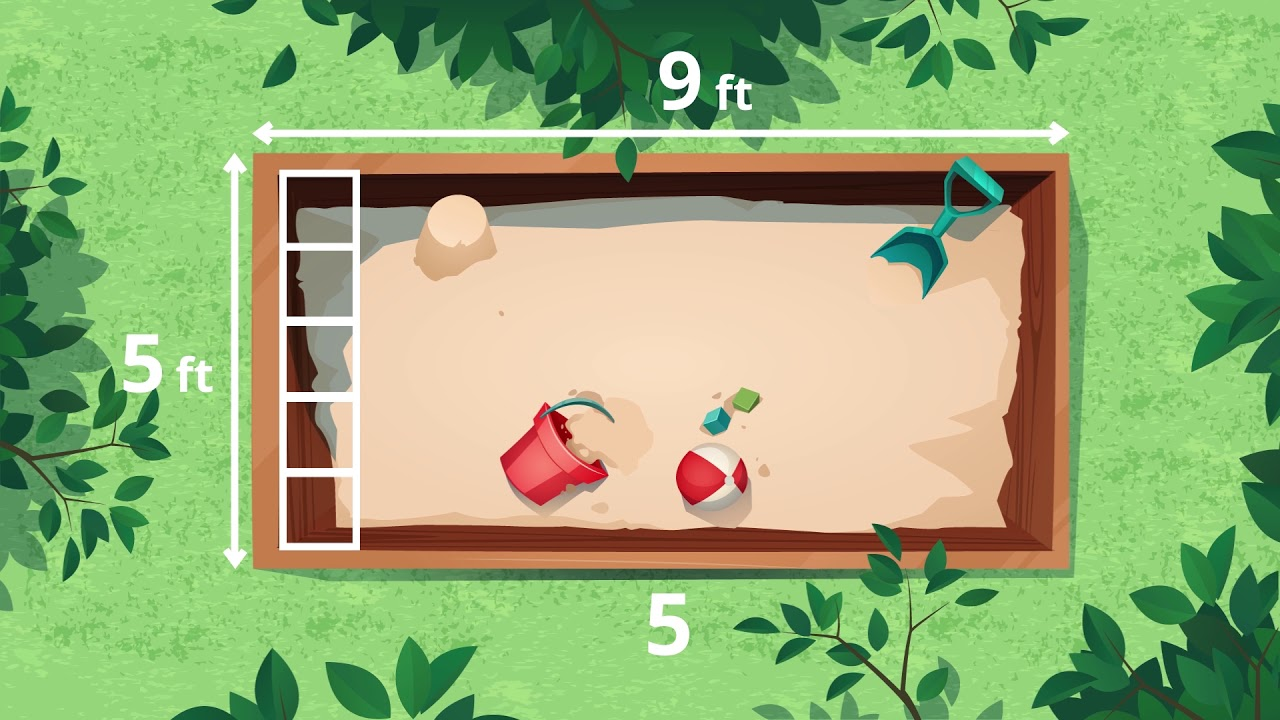By Freckle by Renaissance# Multiplying by 10

##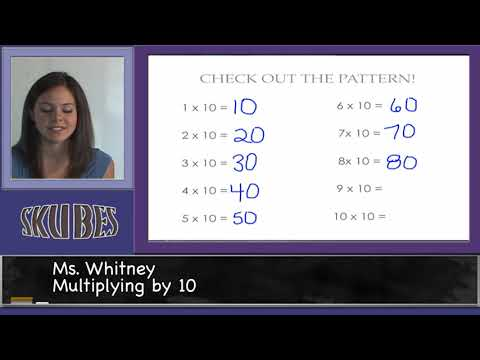By Skubes ed

Multiply one-digit whole numbers by multiples of 10 in the range 10–90 (e.g., 9 × 80, 5 × 60) using strategies based on place value and properties of operations.# [3.MD.7b-1.3] Multiply to find Area - Common Core Standard

##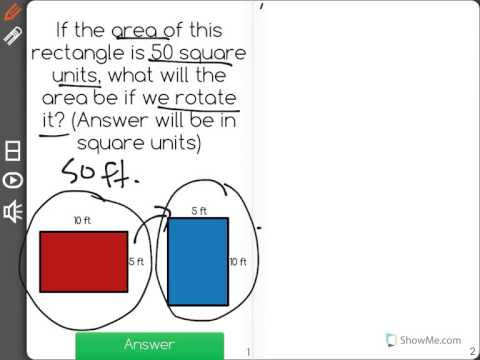By Freckle by Renaissance# Multiplying Mixed Numbers - YourTeacher.com - Pre Algebra Help

##By yourteachermathhelp

For a complete lesson on multiplying mixed numbers go to http://www.yourteacher.com - 1000+ online math lessons featuring a personal math teacher inside every lesson! In this lesson students learn to multiply mixed numbers by first rewriting the mixed numbers as improper fractions then multiplying the improper fractions together then rewriting the resulting improper fraction as a mixed number if necessary. For example to multiply 1 8/15 x 1 1/4 first rewrite the mixed numbers as improper fractions to get 23/15 x 5/4. Next cross-cancel the 15 and 5 to 3 and 1 and we have 23/3 x 1/4. Next multiply across the numerators and denominators to get 23/12. Finally rewrite 23/12 as the mixed number 1 11/12.# Transitioning from counting to multiplying to find area | 3rd grade | Khan Academy

##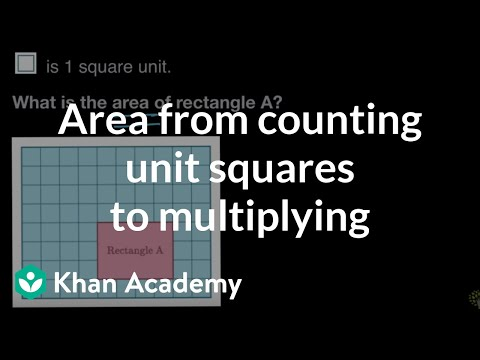By Khan Academy

finds the area of a rectangle both by counting unit squares and multiplying side lengths# Steps to Multiply Big Numbers Using the Standard Method

##By Joseph Gliddon

This video goes step by step though a standard method to multiply a 3 digit number by a 2 digit number. Examples are shown of step by step process for solving multiplication of multi-digit numbers.# Multiply binomials by polynomials

##By Khan Academy

Sal expresses the area of a rectangle whose height is y^2-6y and width is 3y^2-2y+1.